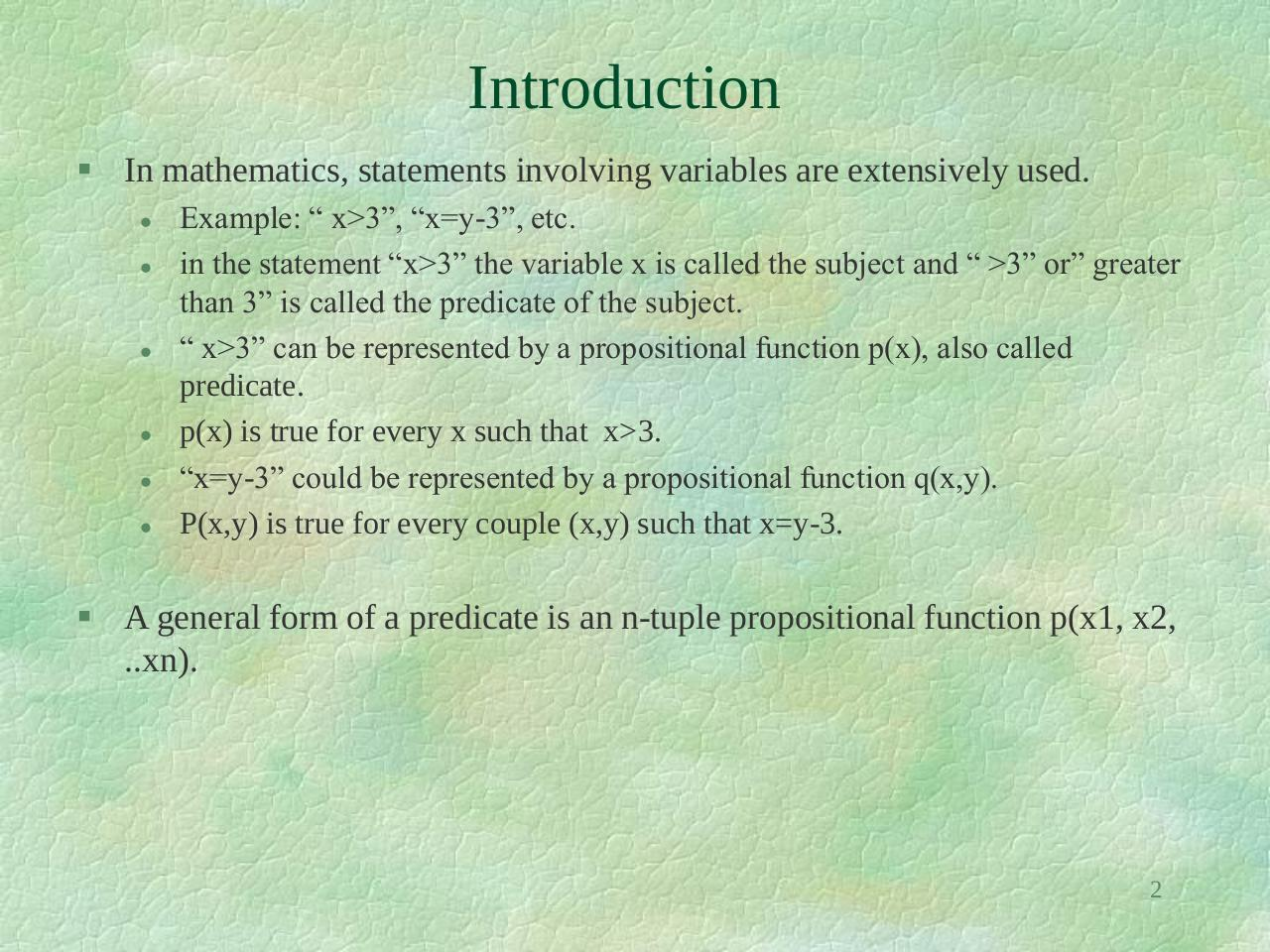Discreet lec 3.pdfPage 1 2 3 4 5 6 7 8 9 10

Text preview

Introduction
 In mathematics, statements involving variables are extensively used.

Example: “ x&gt;3”, “x=y-3”, etc.
in the statement “x&gt;3” the variable x is called the subject and “ &gt;3” or” greater
than 3” is called the predicate of the subject.
“ x&gt;3” can be represented by a propositional function p(x), also called
predicate.
p(x) is true for every x such that x&gt;3.
“x=y-3” could be represented by a propositional function q(x,y).
P(x,y) is true for every couple (x,y) such that x=y-3.

 A general form of a predicate is an n-tuple propositional function p(x1, x2,
..xn).

2